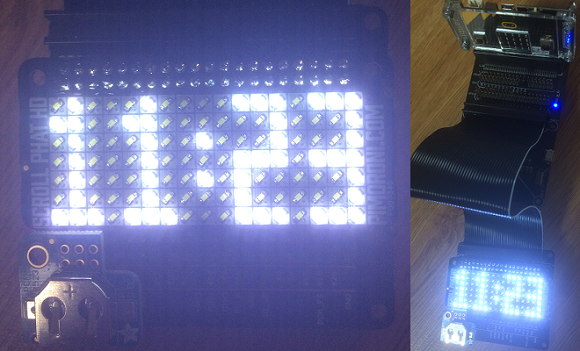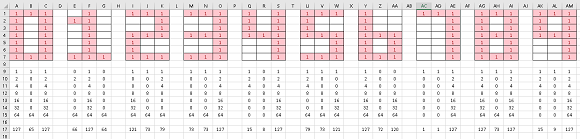# BBC micro:bit Scroll pHAT HD

## Introduction

The Scroll pHAT HD is a raspberry Pi accessory made by the UK electronics company Pimoroni. It consists of 119 LEDs arranged in a 17x7 grid and an IS31FL3731 driver chip all crammed into the pHAT form factor.The LEDs are very bright and there's a lot of them. There's no buffer and you can write very quickly to the display. The board is very well designed and now comes in different coloured versions.

## Connecting

I used a 4tronix Bit:2:Pi as you see in the image. I also used a Mini Black Hat Hack3r, another excellent Pimoroni board and the Adafruit PiRTC to knock up a clock.

## Programming

The pixels are not connected in the order you might want to use. The Pimoroni library has a workaround but I wanted the display to be inverted vertically.

```from microbit import *

class Matrix:
def __init__(self):
self.wreg(0x0A,0,1)
self.wreg(0x0A,1,1)
self.wreg(0,0,1)
self.wreg(1,0,1)
self.fill(0)
for f in range(8):
for i in range(18):
self.wreg(i,0xff)
self.wreg(0x06,0,1)

def wreg(self,r,v,c=0):
if c:
i2c.write(0x74, b'\xFD\x0B')
else:
i2c.write(0x74, b'\xFD\x00')
i2c.write(0x74, bytes([r,v]))

def fill(self, value):
i2c.write(0x74, b'\xFD\x00')
for i in range(6):
d = bytearray([0x24 + i * 24]) + bytearray(([value]*24))
i2c.write(0x74, d, repeat=False)

def set_xy(self,x,y,b):
y = 6 - y
if x > 8:
x = x - 8
y = 6 - (y + 8)
else:
x = 8 - x
led = x * 16 + y
self.wreg(0x24+led,b)

def set_led(self,led,b):
self.set_xy(led%17,led//17,b)

a = Matrix()

# by xy
for y in range(7):
for x in range(17):
a.set_xy(x,y,128)
sleep(50)

sleep(1000)
a.fill(0)

# by LED number
for i in range(119):
a.set_led(i,128)
sleep(50)
```

## Scrolling Text

The Scroll pHAT HD and its discontinued non-HD cousin are intended for scrolling text. The micro:bit font is accessible but a little too small to look good on the display so a font needs to be defined and stored. This takes up a fair bit of space in a script.

The odd order of the LEDs makes it a bit tricky to write the display in one go, unless you are writing the same value to all pixels. That means redrawing the whole display, LED by LED each time the message scrolls. That's a bit blinky, but it's what I did.

The font is stored as a bytearray. I added some line breaks in the definition to fit it onto this page. You should remove them.

```from microbit import *

class Matrix:
def __init__(self):
self.wreg(0x0A,0,1)
self.wreg(0x0A,1,1)
self.wreg(0,0,1)
self.wreg(1,0,1)
self.fill(0)
for f in range(8):
for i in range(18):
self.wreg(i,0xff)
self.wreg(0x06,0,1)

def wreg(self,r,v,c=0):
if c:
i2c.write(0x74, b'\xFD\x0B')
else:
i2c.write(0x74, b'\xFD\x00')
i2c.write(0x74, bytes([r,v]))

def fill(self, value):
i2c.write(0x74, b'\xFD\x00')
for i in range(6):
d = bytearray([0x24 + i * 24]) + bytearray(([value]*24))
i2c.write(0x74, d, repeat=False)

def set_xy(self,x,y,b):
y = 6 - y
if x > 8:
x = x - 8
y = 6 - (y + 8)
else:
x = 8 - x
led = x * 16 + y
self.wreg(0x24+led,b)

def set_led(self,led,b):
self.set_xy(led%17,led//17,b)

def msg(self,m):
font = bytearray(b'\x00\x00\x00\x00\x00\x00\x00\x5f\x00\x00\x00\x07\x00\x07\x00\x14
\x7f\x14\x7f\x14\x24\x2a\x7f\x2a\x12\x23\x13\x08\x64\x62\x36\x49\x55\x22\x50\x00\x05
\x03\x00\x00\x00\x1c\x22\x41\x00\x00\x41\x22\x1c\x00\x14\x08\x3e\x08\x14\x08\x08\x3e
\x08\x08\x00\x50\x30\x00\x00\x08\x08\x08\x08\x08\x00\x60\x60\x00\x00\x20\x10\x08\x04
\x02\x3e\x51\x49\x45\x3e\x00\x42\x7f\x40\x00\x42\x61\x51\x49\x46\x21\x41\x45\x4b\x31
\x18\x14\x12\x7f\x10\x27\x45\x45\x45\x39\x3c\x4a\x49\x49\x30\x01\x71\x09\x05\x03\x36
\x49\x49\x49\x36\x06\x49\x49\x29\x1e\x00\x36\x36\x00\x00\x00\x56\x36\x00\x00\x08\x14
\x22\x41\x00\x14\x14\x14\x14\x14\x00\x41\x22\x14\x08\x02\x01\x51\x09\x06\x32\x49\x79
\x41\x3e\x7e\x11\x11\x11\x7e\x7f\x49\x49\x49\x36\x3e\x41\x41\x41\x22\x7f\x41\x41\x22
\x1c\x7f\x49\x49\x49\x41\x7f\x09\x09\x09\x01\x3e\x41\x49\x49\x7a\x7f\x08\x08\x08\x7f
\x00\x41\x7f\x41\x00\x20\x40\x41\x3f\x01\x7f\x08\x14\x22\x41\x7f\x40\x40\x40\x40\x7f
\x02\x0c\x02\x7f\x7f\x04\x08\x10\x7f\x3e\x41\x41\x41\x3e\x7f\x09\x09\x09\x06\x3e\x41
\x51\x21\x5e\x7f\x09\x19\x29\x46\x46\x49\x49\x49\x31\x01\x01\x7f\x01\x01\x3f\x40\x40
\x40\x3f\x1f\x20\x40\x20\x1f\x3f\x40\x38\x40\x3f\x63\x14\x08\x14\x63\x07\x08\x70\x08
\x07\x61\x51\x49\x45\x43\x00\x7f\x41\x41\x00\x02\x04\x08\x10\x20\x00\x41\x41\x7f\x00
\x04\x02\x01\x02\x04\x40\x40\x40\x40\x40\x00\x01\x02\x04\x00\x20\x54\x54\x54\x78\x7f
\x48\x44\x44\x38\x38\x44\x44\x44\x20\x38\x44\x44\x48\x7f\x38\x54\x54\x54\x18\x08\x7e
\x09\x01\x02\x0c\x52\x52\x52\x3e\x7f\x08\x04\x04\x78\x00\x44\x7d\x40\x00\x20\x40\x44
\x3d\x00\x7f\x10\x28\x44\x00\x00\x41\x7f\x40\x00\x7c\x04\x18\x04\x78\x7c\x08\x04\x04
\x78\x38\x44\x44\x44\x38\x7c\x14\x14\x14\x08\x08\x14\x14\x18\x7c\x7c\x08\x04\x04\x08
\x48\x54\x54\x54\x20\x04\x3f\x44\x40\x20\x3c\x40\x40\x20\x7c\x1c\x20\x40\x20\x1c\x3c
\x40\x30\x40\x3c\x44\x28\x10\x28\x44\x0c\x50\x50\x50\x3c\x44\x64\x54\x4c\x44\x00\x08
\x36\x41\x00\x00\x00\x7f\x00\x00\x00\x41\x36\x08\x00\x10\x08\x08\x10\x08\x00\x00\x00
\x00\x00')
txt = bytearray()
for d in m:
a = (ord(d) - 32) * 5
for i in range(5):
txt.append(font[i+a])
txt.append(0x00)
for i in range(16): txt.append(0x00)
return txt

def scroll(self,t):
txt = self.msg(t)
stt = 0
stp = 16
if len(txt)<16: stp = len(txt)
while stp<len(txt):
for i in range(17):
bts = [(txt[i+stt] >> j & 1) for j in range(7)]
for y in range(7):
self.set_xy(i,y,bts[y]*128)
sleep(5)
stt+=1
stp+=1

a = Matrix()
```

Although there is a small delay in the scrolling loop, the timing is mainly determined by how long it takes interpreted MicroPython to perform the updates the way I have programmed them. It is a bit slow but working after a fashion. Work out how to write encode an entire frame and this will work much better.

## Clock Display

I designed a font for the numerical digits to display a clock. I did this before for an Adafruit display that uses the same driver. The Pimoroni board has more rows, so I made a 3x7 font for the digits to be able to fit 4 of them.Each of the 4 digits needed for the time is 3 columns wide, with a space after each digit and 3 columns for the colon. Thet's just enough for 24 hour time.

```from microbit import *

class Matrix:
def __init__(self):
self.wreg(0x0A,0,1)
self.wreg(0x0A,1,1)
self.wreg(0,0,1)
self.wreg(1,0,1)
self.fill(0)
for f in range(8):
for i in range(18):
self.wreg(i,0xff)
self.wreg(0x06,0,1)

def wreg(self,r,v,c=0):
if c:
i2c.write(0x74, b'\xFD\x0B')
else:
i2c.write(0x74, b'\xFD\x00')
i2c.write(0x74, bytes([r,v]))

def fill(self, value):
i2c.write(0x74, b'\xFD\x00')
for i in range(6):
d = bytearray([0x24 + i * 24]) + bytearray(([value]*24))
i2c.write(0x74, d, repeat=False)

def set_xy(self,x,y,b):
y = 6 - y
if x > 8:
x = x - 8
y = 6 - (y + 8)
else:
x = 8 - x
led = x * 16 + y
self.wreg(0x24+led,b)

def set_led(self,led,b):
self.set_xy(led%17,led//17,b)

def set_dig(self,c,d):
x = [0,4,10,14][c]
ds = [127,65,127,66,127,64,121,73,79,73,73,127,15,8,
127,79,73,121,127,72,120,1,1,127,127,73,127,15,9,127]
for i in range(3):
v = ds[d*3+i]
bts = [(v >> j & 1) for j in range(7)]
for y in range(7):
self.set_xy(x+i,y,bts[y]*128)

def bcd2bin(value):
return (value or 0) - 6 * ((value or 0) >> 4)

def get_time():
i2c.write(0x68, b'\x03')
m = bcd2bin(buf)
h = bcd2bin(buf)
return m,h

a = Matrix()
lastm,lasth = get_time()
a.set_dig(0,lasth//10)
a.set_dig(1,lasth%10)
a.set_xy(8,2,128)
a.set_xy(8,5,128)
a.set_dig(2,lastm//10)
a.set_dig(3,lastm%10)
while True:
mm,hh = get_time()
if mm!=lastm or hh!=lasth:
a.set_dig(0,hh//10)
a.set_dig(1,hh%10)
a.set_dig(2,mm//10)
a.set_dig(3,mm%10)
lastm = mm
lasth = hh
sleep(1000)
```

Depending on the way I power the circuit, I sometimes get an error reading this particular RTC, not just with this circuit too. You can use another RTC if you get the same problem or code for it with a try block.

## Challenges

1. Design a 5x3 font for RTC digits and use the spare LEDs to display binary time or tick through the seconds.
2. Add the inputs needed to make the clock usable. Display the date and code for some alarms.
3. Trim down the core class as much as you like and use the display to make a game.
4. Forget about the RTC. You can show 4 digit numbers comfortably so have a display for analog readings or accelerometer values. Adapt the method for displaying a digit so that it displays an integer value from -999 to 9999.
5. Adapt the code for the clock so that the digits are smoothed with different PWM values around the edges.